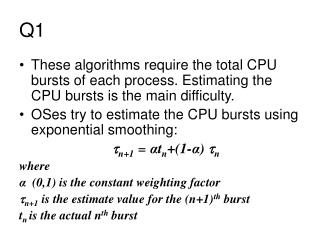DownloadDownload PresentationP1 – BLOCKED P2 – BLOCKED P3 – BLOCKED/SUSPEND P4 – RUNNING P5 – READY

# P1 – BLOCKED P2 – BLOCKED P3 – BLOCKED/SUSPEND P4 – RUNNING P5 – READY

Download Presentation## P1 – BLOCKED P2 – BLOCKED P3 – BLOCKED/SUSPEND P4 – RUNNING P5 – READY

- - - - - - - - - - - - - - - - - - - - - - - - - - - E N D - - - - - - - - - - - - - - - - - - - - - - - - - - -
##### Presentation Transcript

1. Q1 • These algorithms require the total CPU bursts of each process. Estimating the CPU bursts is the main difficulty. • OSes try to estimate the CPU bursts using exponential smoothing: n+1 = αtn+(1-α) n where α (0,1) is the constant weighting factor n+1is the estimate value for the (n+1)th burst tn is the actual nth burst

2. Q2 • Even in multiprogramming environment, it is found that CPU is idle most of the time. This is because, I/O operations are much slower than computations ant it is possible that at a given time, all processes are in the blocked state. • Suspended state is created to increase CPU utilization by swapping out blocked processes and making way for other processes to come in main memory (either from suspended state or a new process is honored) • Bringing in a process from the suspended state may not help as it may still not be ready to use the processor • It is better to split the suspended state into blocked/suspend and ready/suspend. • Whenever swapping is done, we bring in a new process or we bring in a process from the ready/suspend state

3. Q3 • P1 – BLOCKED • P2 – BLOCKED • P3 – BLOCKED/SUSPEND • P4 – RUNNING • P5 – READY Demand Paging – P2, P3, & P5

4. Q4 MLF (4 queues with q=2i (solu. 1) 5 0 10 15 20 25 1 2 3 4 5 Av. WT = (12+12+15+15+7)/5 = 61/5 =12.2 Av. TAT = (17+16+23+24+10)/5 = 90/5 = 18

5. Q4 MLF (4 queues with q=2i (solu. 2) 5 0 10 15 20 25 1 2 3 4 5 Av. WT = (12+12+15+15+6)/5 = 60/5 =12.0 Av. TAT = (17+16+23+24+9)/5 = 89/5 = 17.8

6. Q5 (a) First-Come-First-Served (FCFS) 5 0 10 15 20 1 2 3 4 5 AWT = (6+1+13+4+7)/5 = 31/5 = 6.2 NTAT = 13/7+5/4+18/5+7/3+12/6 =

7. Q5 (b) Round Robin (q=3) 5 0 10 15 20 1 2 3 4 5 AWT = (5+8+11+3+9)/5 = 36/5 = 7.2 NTAT = 12/7+12/4+16/5+6/3+14/5 =

8. Q5 (c) Virtual Round Robin (q=3) 5 0 10 15 20 1 2 3 4 5 AWT = (11+8+5+4+9)/5 = 37/5 = 7.4 NTAT = 18/7+12/4+10/5+7/3+14/5 =